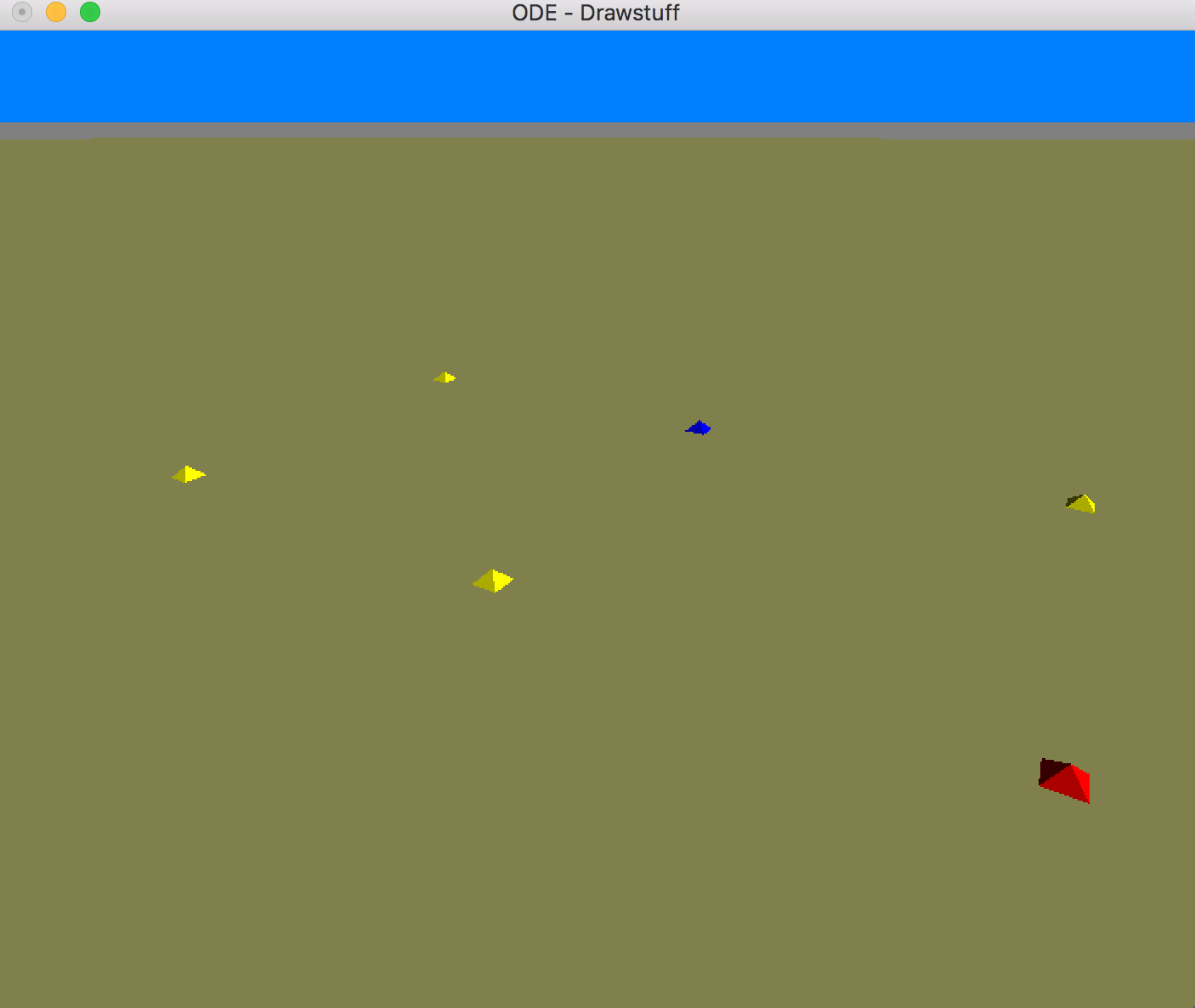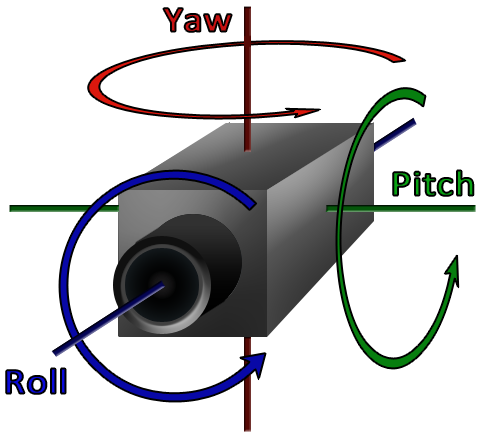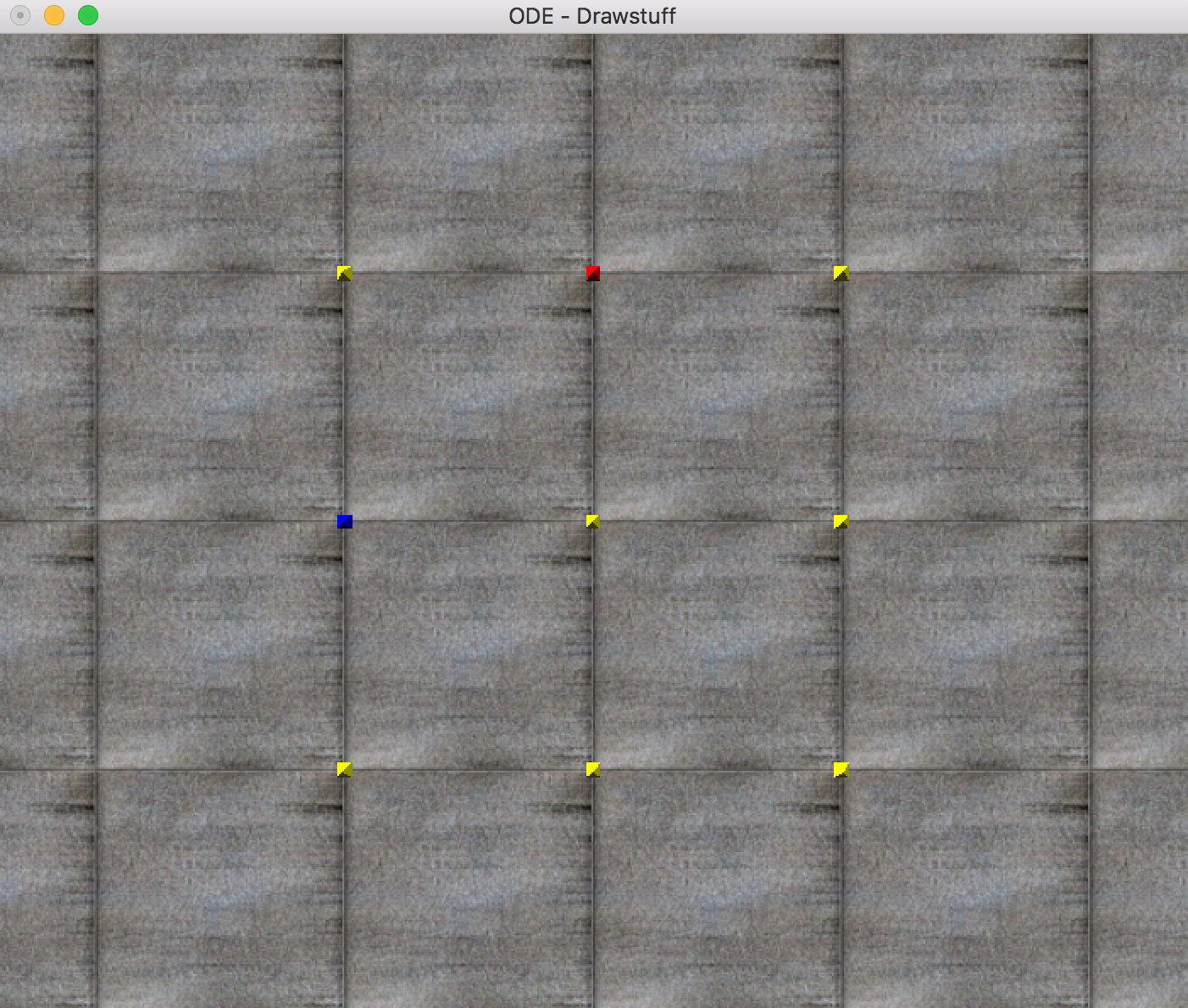# Simulator Settings¶

## Empty Simulator¶

Let’s start by just making an empty simulation. The following python code opens up an empty simulation and runs it for a few seconds before exiting.

#creates simulator object
sim = pyrosim.Simulator()

#starts simulation
sim.start()


This is what the simulator looks like when it is empty.Headless running means the physics will run. but the graphics will not render. This means simulation will finish much faster. You can run headless by setting play_blind to True.

sim = pyrosim.Simulator(play_blind=True)
sim.start()


## Timing Parameters¶

By default, the simulation runs for 100 discrete times steps with a time step interval dt=0.05 seconds. We can increase or decrease the number of time steps by changing the parameter eval_time. The following code runs the same empty simulation but for 1,000 time steps at half the time step interval.

sim = pyrosim.Simulator(eval_time=1000, dt=0.025)
sim.start()


The simulator should now run longer because there are now 10x more frames being drawn and the current rendering system waits a fixed time before rendering the frame.

NOTE: When running actual robots, changing dt will most likely change the outcome of the simulation. In general, a lower dt provides a more stable, yet slower, simulation.

## Camera Parameters¶

You can set the initial camera position of the simulation by changing its position (xyz) and its heading (also called yaw), pitch, and roll (hpr). hpr values are in degrees. ODE uses a Z-up coordinate system. This means the z axis is in the ‘up’ direction.The following sets the camera at position (0,0,5) looking straight at the ground (0,-90,0). You can also use the default textures provided by ODE’s rendering package by altering use_textures

sim = pyrosim.Simulator(xyz=[0,0,5], hpr=[0,-90,0], use_textures=True)
sim.start()


This should return an initial view that looks like this:The red dot (top of the image) is in the +x direction and the blue dot (left side of the image) is in the +y direction.